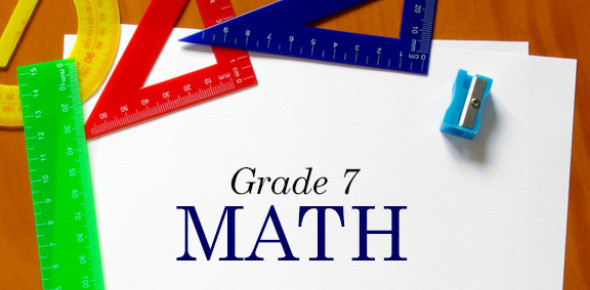# Grade 7 Math Test Online Quiz!

10 Questions | Attempts: 80645SettingsMaths is a challenging subject for most children. How good are you with Maths? To see how well you score in this subject, take this amazing quiz. It will test your essential mathematical skills in division, multiplication, fractions, decimals, Percents, and ratios. Please read the questions carefully and answer. So, let's give it a try. All the best!

• 1.
Name a equation that makes (+6), by using these numbers: (+9) (+7) (+2) (-8) (-3) (-1) !!
• A.

(+9) + (-1) = (+6)

• B.

(+9) + (-3) = (+6)

• C.

(+7) + (-3) = (+6)

• D.

(+7) + (-8) = (+6)

• 2.
What represents (-4)
• A.

(+) (-) (+) (+) (+) (+)

• B.

(-) (-) (+) (+)

• C.

(+) (-) (+) (-) (-) (-) (-) (-)

• D.

(-) (+) (-) (-) (-)

• 3.
Calculate: (-8) + (+2) - (-6) = ?
• A.

(-12)

• B.

(0)

• C.

(+4)

• D.

(+16)

• 4.
What is the percentage of 3/20?
• A.

3%

• B.

12%

• C.

15%

• D.

60%

• 5.
What number goes in the blank? _/5 - 7/10 = 1/10
• A.

3

• B.

4

• C.

5

• D.

8

• E.

12

• 6.
What does 2/3 + 1/4 = ?
• A.

11/24

• B.

3/7

• C.

11/12

• D.

1 whole

• 7.
What does decimal is 7%?
• A.

0.07

• B.

0.7

• C.

7

• D.

70

• E.

700

• 8.
Evaluate for X:  -5 = X + 7
• A.

2

• B.

-2

• C.

12

• D.

-12

• 9.
If the length and breadth of a rectangle are 36 cm and 24 cm, its perimeter will be__
• A.

140 cm

• B.

120 cm

• C.

864 cm

• 10.
3 + 4b = 15. What is the value of b in this equation?
• A.

6

• B.

3

• C.

8

## Related TopicsBack to top
×

Wait!
Here's an interesting quiz for you.